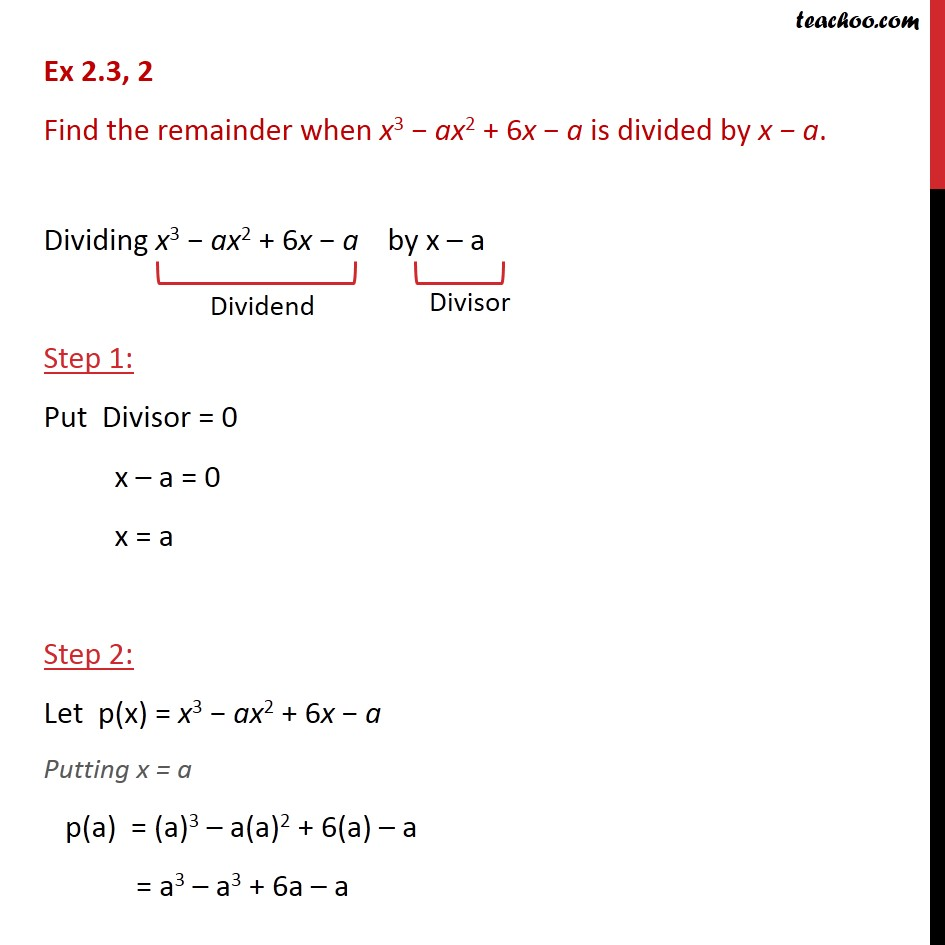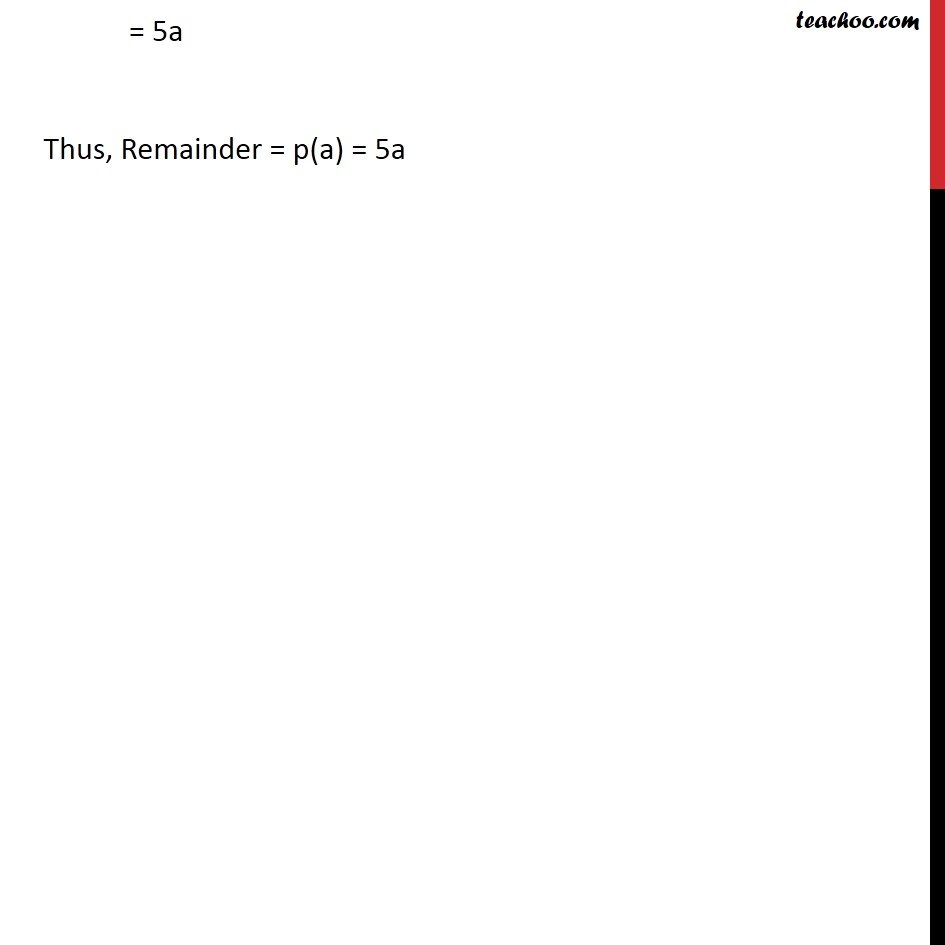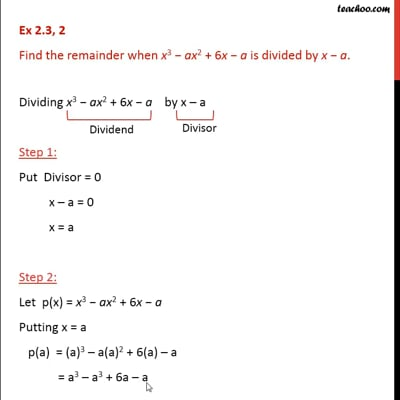Remainder Theoram

Chapter 2 Class 9 Polynomials
Concept wiseThis video is only available for Teachoo black users

Introducing your new favourite teacher - Teachoo Black, at only ₹83 per month

### Transcript

Ex 2.3, 2 Find the remainder when x3 − ax2 + 6x − a is divided by x − a. Dividing x3 − ax2 + 6x − a by x – a Step 1: Put Divisor = 0 x – a = 0 x = a Step 2: Let p(x) = x3 − ax2 + 6x − a Putting x = a p(a) = (a)3 – a(a)2 + 6(a) – a = a3 – a3 + 6a – a = 5a Thus, Remainder = p(a) = 5a = (−125)/8 + 3(25/4) – 15/2 + 1 = (−125)/8 + 75/4 – 15/2 + 1 = (−125 + 75(2) − 15(4) + 1(8) )/8 = (−125 + 150 − 60 + 8)/8 = (−27)/8 Thus, remainder = p((−5)/2) = (−27)/8